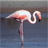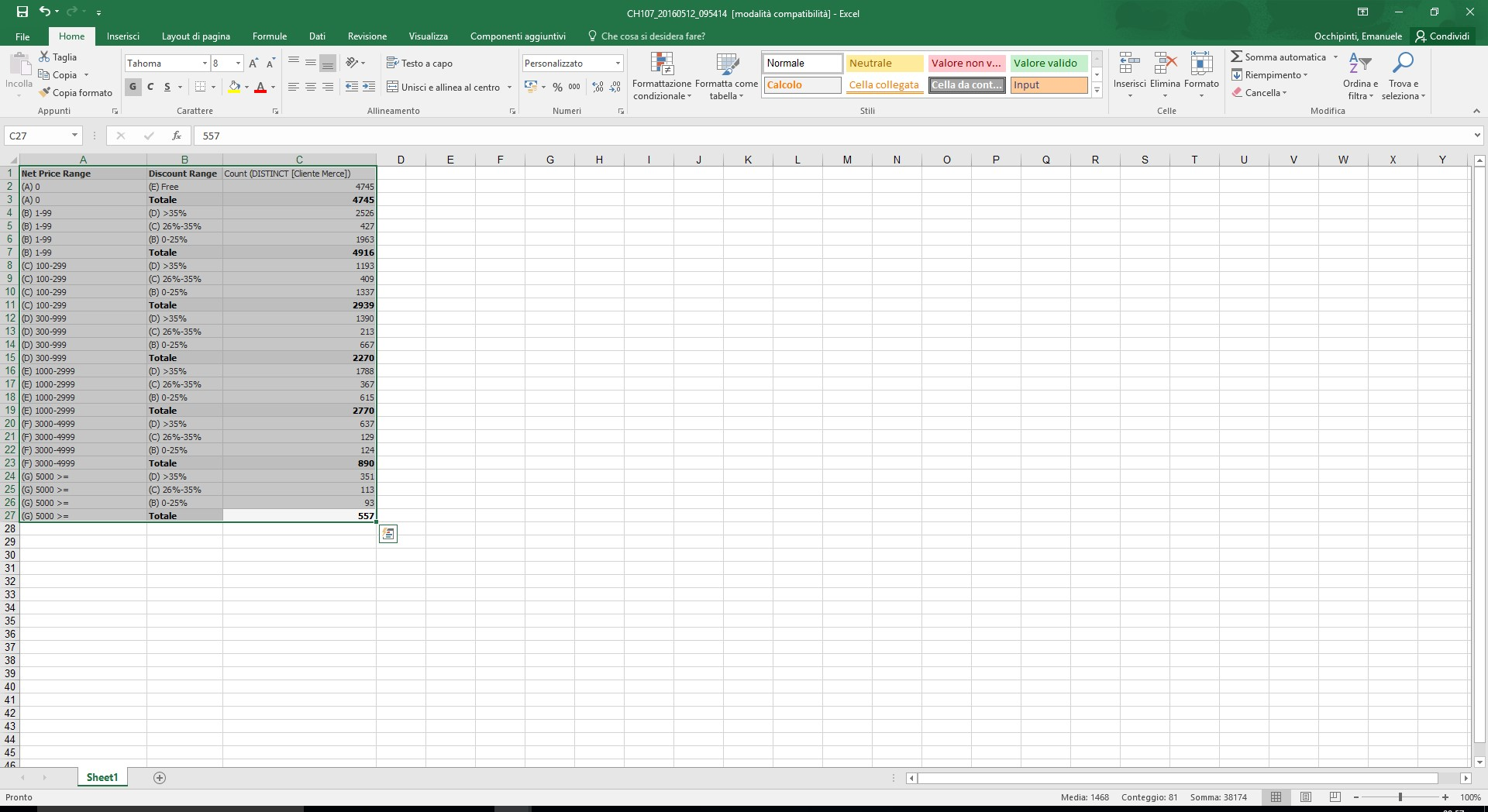# QlikView App Dev

Discussion Board for collaboration related to QlikView App Development.

Announcements
Customer & Partners, DEC. 9, 11 AM ET: Qlik Product & Strategy Roadmap Session: Data Analytics REGISTER NOW
cancel
Showing results for
Did you mean:Not applicable

## Calculated DImension IF Sum

Hi Everybody,

I have the following dataset:

SalesOrder

CustomerID

NetPrice

Discount

PriceList

I have first to aggregate:

- NetPrice at Customer level

- Discount at Customer Level

- PriceList at Customer Level

then define NetPrice Ranges , e.g. 2 ranges A and B:

A = NetPrice @ Customer Level <= 1000

B = NetPrice @ Customer Level > 1000

then define Discount Ranges , e.g. 3 ranges D1 D2 D3 as follows:

D1 = Discount @ Customer Level / PriceList @ CUstomer Level <= 10%

D2 = Discount @ Customer Level / PriceList @ CUstomer Level <= 30%

D3 = Discount @ Customer Level / PriceList @ CUstomer Level > 30%

At the end I need to count distinct Customer for each NetPriceRanges and Discount Ranges

Can anybody help me?

Emanuele

1 Solution

Accepted SolutionsMVP & Luminary

For the NetPrice ranges you could do something like this:

if(aggr(sum(NetPrice), CustomerID)<=1000, 'A', 'B')

or maybe in another clustered way with a variable cluster-size:

class(aggr(sum(NetPrice), CustomerID), \$(vClusterSize))

The discount ranges could be calculated in a similar way:

if(aggr(sum(Discount)/sum(PriceList), CustomerID)<=0.1, 'D1',

if(aggr(sum(Discount)/sum(PriceList), CustomerID)<=0.3,'D2', 'D3'))

Very probably you will need further dimensions within the aggr-part, for example Month and Year.

- Marcus

7 RepliesMVPMVP & Luminary

For the NetPrice ranges you could do something like this:

if(aggr(sum(NetPrice), CustomerID)<=1000, 'A', 'B')

or maybe in another clustered way with a variable cluster-size:

class(aggr(sum(NetPrice), CustomerID), \$(vClusterSize))

The discount ranges could be calculated in a similar way:

if(aggr(sum(Discount)/sum(PriceList), CustomerID)<=0.1, 'D1',

if(aggr(sum(Discount)/sum(PriceList), CustomerID)<=0.3,'D2', 'D3'))

Very probably you will need further dimensions within the aggr-part, for example Month and Year.

- MarcusMVP

Try below expressions

1) Count of Net Price Range A

= Count({<CustId ={"=sum(NetPrice)<=1000"}>} CustId )

2) Count of Net Price Range B

= Count({<CustId ={"=sum(NetPrice)>1000"}>} CustId )

3) Discount Range D1

= Count({<CustId ={"=sum(Discount)/Sum(Pricelist)<=0.1"}>} CustId )

4) Discount Range D2

= Count({<CustId ={"=sum(Discount)/Sum(Pricelist)<=0.3"}>} CustId )

5) Discount Range D3

= Count({<CustId ={"=sum(Discount)/Sum(Pricelist)>0.3"}>} CustId )

You can add the the Dimensions you needCreator II

Hi, hope the attached helps.Not applicable
Author

Thanks it works!Not applicable
AuthorI get this kind of result. Now I need to express in termf of % on total disctinct customer at Net Price Range Level.

For instance the NetPriceRange  (B) and Disc Range (B) -> 2526 as 2526/4916 (where 4916 is the Total distinct customer for the NetPrice range B. I tried with Total Qualifier but I do not know how to indicate the calculated dimension "Net Price Range"

Thanks a lot

EmanueleMVP & Luminary

A global TOTAL will work but if you need a TOTAL on a certain dimension-level you couldn't do it with a calculated dimension. In this case you need to use an adjusted version of the expression from the calculated dimension which returned for each dimension-value the TOTAL of the expression which you then used as divisor - but this could be quite complicated and will be rather slow especially by larger datasets.

If this won't needed to be absolutely flexible of any selections it could be better to calculate these cluster within the script - similar to the suggestion from anagharao.

- MarcusCommunity Browser